## residual sum of squares in Nonlinear curve...

Hello!

How to calculate the residual sum of squares of a Nonlinear Fitting?

I tried but was unsuccessful.## express the function z in terms of Legendre functi...

How I can prove the following equation in red box.

Also, Pn(v) and qn(v) are the real combinations of half-integer Legendre functions.

https://math.stackexchange.com/questions/2746660/potential-flow-around-a-torus-laplace-equation-in-toroidal-coordinates/3809487#3809487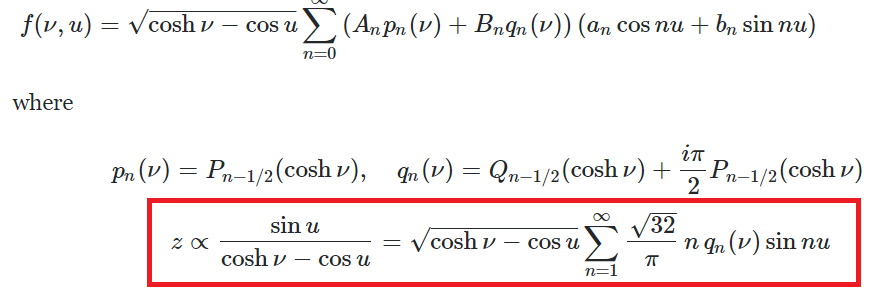## How is this Basisgrid procedure programmed?...

I did already some programmingexercises , but this sort of programming example i did not encounter in the programmingbook
How it is programmed in general lines ?
I do have a lot of small procedures to learn from

But it is too much asked here on the forum to explain all ins and outs for this procedure, because it is not that simple.

## Changing the standard unit to Joules per kilogram ...

When I'm doing math more complicated, than in the first line, Maple gives the incorrect type of unit.
I want the unit as displayed in the first line, but by using the math/syntax shown in the last line.

Maple does not have "Joule per kilogram times kelvin", as a choosable option when I do the syntax in the last line.

Is there any way I can end up with the correct unit, even tho I am using syntaxes?
In theory, I should end up with the same answer as a result?.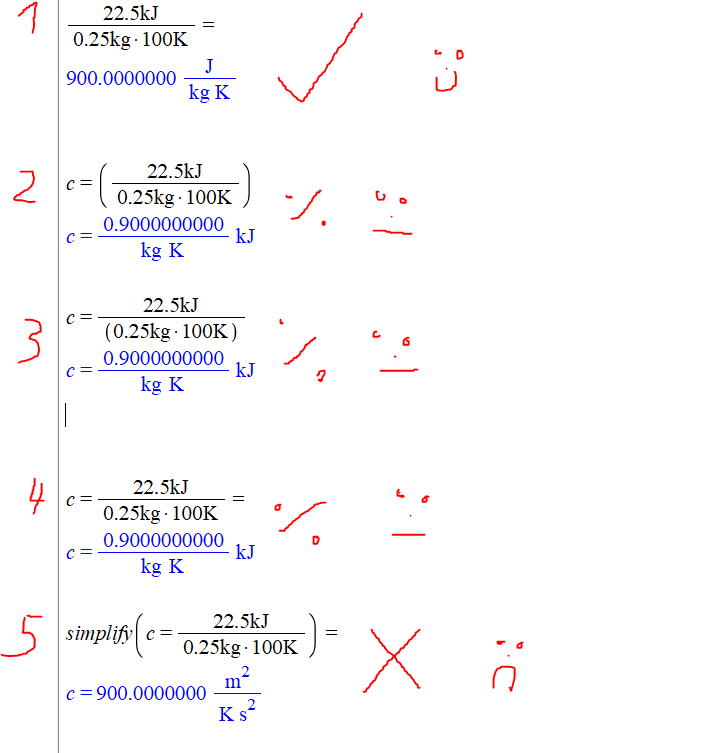## Maple 2D Output automatic aligment...

Is it possible to make executed math aka 2D Outputs, automatically align left?
I'm doing it manually for every execution I make. But is there any setting, that does orient the text to the left by standard?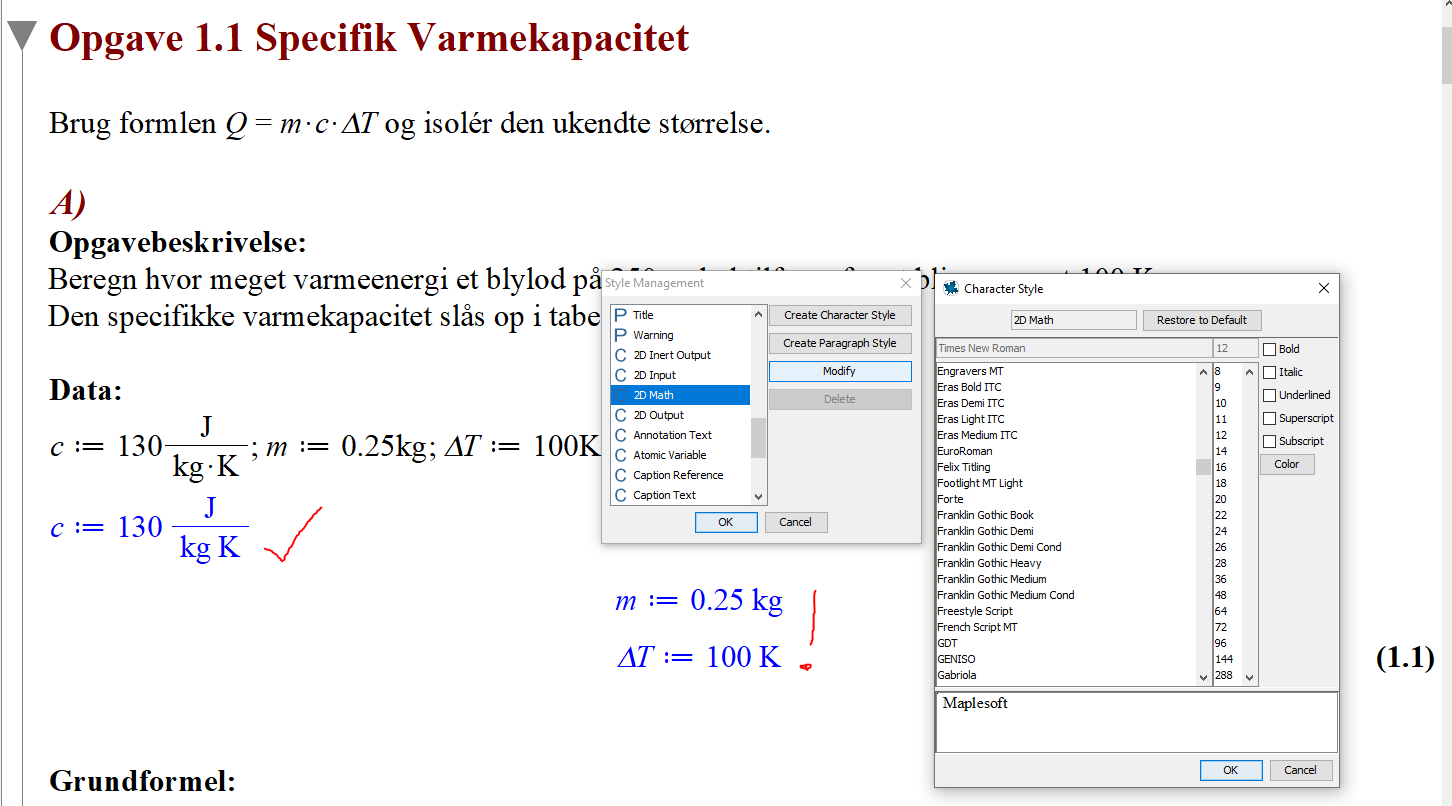## How to Perform Set Operations with Intervals?...

I have intervals A=(-infinity,7), B=(-10,25), and C=(15,infinity). How do I use Maple to perform the union and intersection of such intervals?

## new issue using Physics:-Latex() . Changes typeset...

I spend all day on this, since some tests I have were failing when I changed to using Physics:-Latex() vs. latex(). I made no change at all in my own code other than to replace latex() by Physics:-Latex().

I am still not sure why that is, but it seems due to symbol clash somewhere.  First time I run my test, it passes, next time if fails. When I change back to latex(), the test passes each time.

This seems to be due to when calling Physics:-Latex() the very first time, it automatically changing diff(y(x),x) to y'(x). May be it is storing its own x in there. (this happens on special input and not for each input, that is why only some tests fail and not all of them).

So next time I call the test it failes. May be due to different x being used. I do not know.

All what I know now is that using latex() makes my dsolve test passes each time.

But here I will show the issue which I think if fixed, should fix the main problem.

Calling Physics:-Latex() causes it to automatically change diff(y(x),x) to y'(x)  even though I am not using Typeseeting at all.

```restart;
s:= y = u*x:  #special case input which causes the typesetting to kick in
ode:=diff(y(x),x)=1:
print(ode);
Physics:-Latex(s,output = string):
print(ode);

```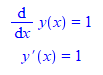Notice how the derivative changes on its own to using y'(x).  Now compare with latex()

```restart;
s:= y = u*x:
ode:=diff(y(x),x)=1:
print(ode);
latex(s,output = string):
print(ode);
```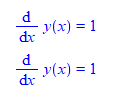So something happens inside Physics:-:Latex which causes this.

For now, I will change back to latex() until I figure more what is going on so that my test do not fail. I like to use Physics:-:Latex as it made the latex look much better.

Maple 2020.1, Physics 778

 > restart;
 > interface(version)> Physics:-Version();> s:= y = u*x: ode:=diff(y(x),x)=1: print(ode); Physics:-Latex(s,output = string): #latex(s,output = string): print(ode);>